# A tensile load of 50,000 lb is applied to a metal bar with a 0.6 in. * 0.6 in.cross section and a gauge length of 2 in. Under this load, the bar elastically deforms so that the gauge length increases to 2.007 in. and the cross section decreases to 0.599 in. * 0.599 in. Determine the modulus of elasticity and Poisson’s ratio for this metal.

Question
1 views

A tensile load of 50,000 lb is applied to a metal bar with a 0.6 in. * 0.6 in.cross section and a gauge length of 2 in. Under this load, the bar elastically deforms so that the gauge length increases to 2.007 in. and the cross section decreases to 0.599 in. * 0.599 in. Determine the modulus of elasticity and Poisson’s ratio for this metal.

check_circle

Step 1

Given:

The tensile load is 50,000 lb.

The area of the metal bar is 0.6 in x 0.6 in.

The gauge length is 2 in.

The increase in the gauge length is 2.007 in.

The decrease in area is 0.599 in x 0.599 in.

Step 2

The formula to calculate the modulus of elasticity is,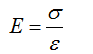The formula to calculate the stress is,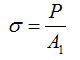The formula to calculate the strain is,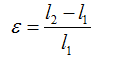Here, P is the load, A1 is the initial area of the bar, l1 is the initial length, and l2 is the final length.

The formula to calculate the Poisson’s ratio is,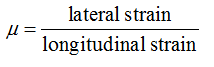Step 3

Calculate the stress.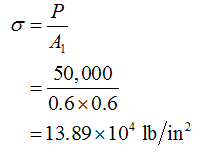Calculate the strain.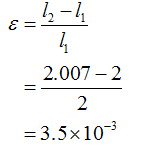Calculate the modulus of elasticity.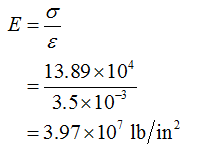...

### Want to see the full answer?

See Solution

#### Want to see this answer and more?

Solutions are written by subject experts who are available 24/7. Questions are typically answered within 1 hour.*

See Solution
*Response times may vary by subject and question.
Tagged in